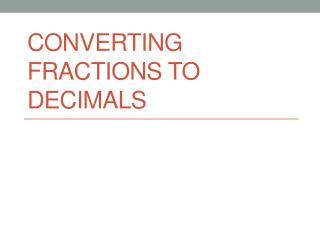DownloadDownload PresentationConverting Fractions to Decimals

# Converting Fractions to Decimals

Télécharger la présentation## Converting Fractions to Decimals

- - - - - - - - - - - - - - - - - - - - - - - - - - - E N D - - - - - - - - - - - - - - - - - - - - - - - - - - -
##### Presentation Transcript

1. Converting Fractions to Decimals

2. “Divided by”For example, means 2 divided by 3, or2 wholes split into 3 equal parts • What does the fraction bar mean?

3. Divide the numerator by the denominator • How do we convert a fraction to decimal form?

4. Memory Trick

5. What isin decimal form?

6. The whole number part (2) is not going to change, so just turn the fraction into a decimal. • What isin decimal form?

7. Collaborative Station • Students will work with their elbow partners unless there needs to be a group of 3. • Each partner will have a sheet of paper with different fractions. • Each round, the partners convert their own fraction into decimal form. Once they are both done, they will compare the decimals to see which is greater.

8. Collaborative Station Example: • For round 1, Partner A has the fraction Partner B has the fraction • Partner A does the work and finds that = 0.5Partner B does the work and finds that = 0.4 • On their work papers, both partners write down: = 0.5 > 0.4 =

9. Independent Station • Each problem will ask you to compare a fraction and a decimal. • Convert the fraction to decimal form, then use the correct symbol (>, <, or =) • On your work paper, show the work for how you converted the fraction